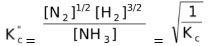# Factors Affecting Equilibrium Constant

## Factors Affecting Equilibrium Constant

(1) Nature of Reactants And/or Products

The value of equilibrium constant depends on the nature of reactants and/or products. By changing the reactant(s) or product(s) of a reaction, its equilibrium constant will change. For example

N2(g) + O2(g)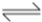2NO(g)

N2(g) + 2O2(g)2NO2(g)

Although the reactants are same but products in the two reactions being different, the value of equilibrium constant for the two reactions will be different.

Similarly for reactions,

H2(g) + I2(g)2HI(g)

H2(g) + Cl2(g)2HCl(g)

the values of the equilibrium constant for the two reactions will be completely different.

(2) Temperature

The variation of equilibrium constant with temperature is given by the relation log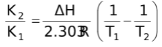.

This can be simply obtained by Arrhenius equation.

Starting with Arrhenius equation of rate constant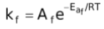….(2)

where, kf = rate constant for forward reaction, Af = Arrhenius constant of forward reaction,

and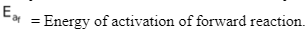= Energy of activation of forward reaction.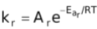….(3)

where, kr = rate constant for backward reaction, Ar = Arrhenius constant of backward reaction and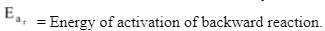= Energy of activation of backward reaction.

Dividing (2) by (3) we get,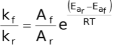We know that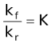(equilibrium constant )

∴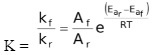At temperature T1,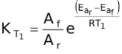….(4)

At temperature T2,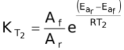….(5)

Dividing (5) by (4) we get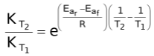Taking log of both the sides,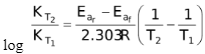The enthalpy of a reaction is defined in terms of activation energies of forward and reverse reactions as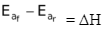= ΔH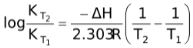∴log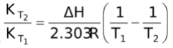….(6)

(a) If ΔH is +ve (endothermic) an increase in temperature (T2 > T1) will make K2 > K1, i.e. the reaction goes more towards the forward direction and vice-versa.

(b) If ΔH is −ve (exothermic) an increase in temperature (T2 > T1), will take K2 < K1 i.e., the reaction goes in the reverse direction.

### (3) Stoichiometry of Reaction

The value of Kp and Kc depends upon the stoichiometry of reaction as

N2(g) + 3H2(g)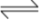2NH3(g)

Kc =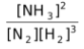….(i)

1/2 N2(g) + 3/2 H2(g)NH3(g)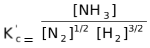….(ii)

From (i) and (ii),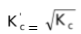### (4) Mode of Writing a Chemical Equation

The value of Kp and Kc also depend on the method of representing a chemical equation.

N2(g) + 3H2(g)2NH3(g)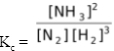If the equilibrium reaction is reversed,

2NH3(g)N2(g) + 3H2(g)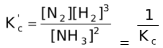Now, if we write the equilibrium reaction as,

NH3(g)1/2 N2(g) + 3/2 H2(g)